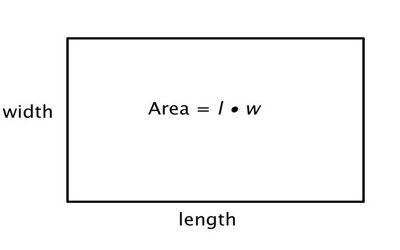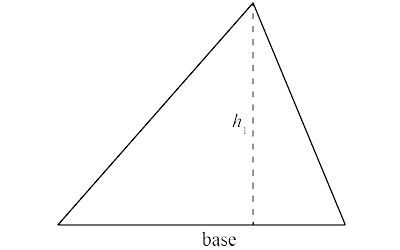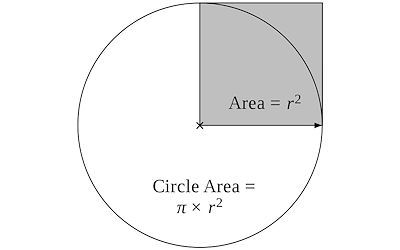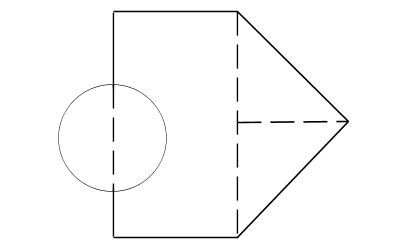### Formulas for Calculating Square Footage

When measuring how many square feet of sod you will need for your landscaping project, it is important to be as accurate as possible. These formulas will help with general area problems you will come across in measuring for installing sod. So, put away those geometry books and follow these simple formulas. You may want to use our custom calculators below.

### Area of aSquare or Rectangle

Area = Length x Width

Example: Area = 20' x 60'

Area = 1,200 square feet### Calculate for a square or rectangle### Area of a Triangle

Area = 0.5 x Base x Height

Example:  Area = 0.5 x 50' x 100'

Area = 2,600 square feet

### Area of a Circle

Area= 3.14 x (Radius x 2 )

Example:  Area = 3.14 x 20' x 20'

Area = 1,250 square feet### Calculate for a circle### Area of unusual shapes

Calculations can be made by totaling different sections. In this case, calculate and add together the area of the triangle and the rectangle, and one half the area of the circle.

You can use our calculators for each individual piece.